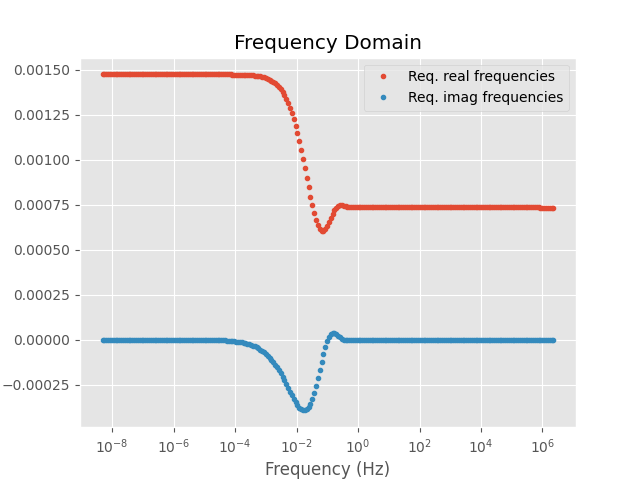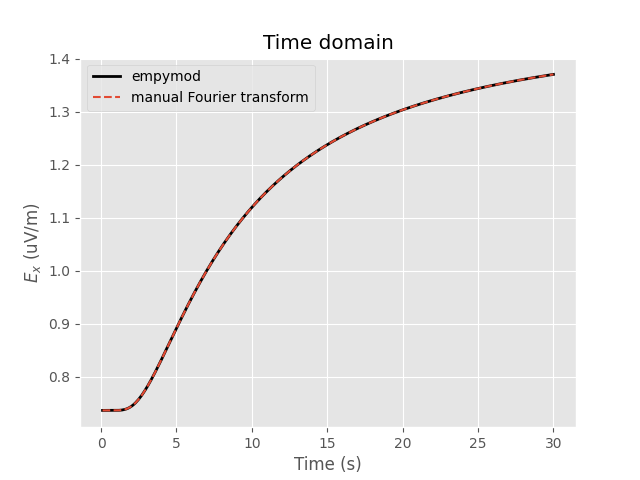# Transform utilities within empymod for other modellers#

This is an example how you can use the Fourier-transform tools implemented in `empymod` with other modellers. You could achieve the same for the Hankel transform.

`empymod` has various Fourier transforms implemented:

• Digital Linear Filters DLF (Sine/Cosine)

• Quadrature with Extrapolation QWE

• Logarithmic Fast Fourier Transform FFTLog

• Fast Fourier Transform FFT

For details of all the parameters see the `empymod`-docs or the function’s docstrings.

```import empymod
import numpy as np
import matplotlib.pyplot as plt
plt.style.use('ggplot')
```

## Model and transform parameters#

The model actually doesn’t matter for our purpose, but we need some model to show how it works.

```# Define model, a halfspace
model = {
'src': [0, 0, 0.001],     # Source at origin, slightly below interface
'rec': [6000, 0, 0.001],  # Receivers in-line, 0.5m below interface
'depth': ,             # Air interface
'res': [2e14, 1],         # Resistivity: [air, half-space]
'epermH': [0, 1],         # Set el. perm. of air to 0 because of num. noise
}

# Specify desired times
time = np.linspace(0.1, 30, 301)

# Desired time-domain signal (0: impulse; 1: step-on; -1: step-off)
signal = 1

# Get required frequencies to model this time-domain result
# => we later need ``ft`` and ``ftarg`` for the Fourier transform.
# => See the docstrings (e.g., empymod.model.dipole) for available transforms
#    and their arguments.
time, freq, ft, ftarg = empymod.utils.check_time(
time=time, signal=signal, ft='dlf', ftarg={}, verb=3)
```
```time        [s] :  0.1 - 30 : 301  [min-max; #]
Fourier         :  DLF (Sine-Filter)
> Filter      :  Key 201 CosSin (2012)
> DLF type    :  Lagged Convolution
```

## Frequency-domain computation#

=> Here we compute the frequency-domain result with `empymod`, but you could compute it with any other modeller.

```fresp = empymod.dipole(freqtime=freq, **model)
```
```:: empymod END; runtime = 0:00:00.029055 :: 1 kernel call(s)
```

Plot frequency-domain result

```plt.figure()

plt.title('Frequency Domain')
plt.plot(freq, 1e9*fresp.real, 'C0.', label='Req. real frequencies')
plt.plot(freq, 1e9*fresp.imag, 'C1.', label='Req. imag frequencies')
plt.legend()
plt.xscale('log')
plt.xlabel('Frequency (Hz)')
plt.ylabel('\$E_x\$ (nV/m)')

plt.show()
```## Fourier transform#

```# Compute corresponding time-domain signal.
tresp, _ = empymod.model.tem(
fEM=fresp[:, None],
off=np.array(model['rec']),
freq=freq,
time=time,
signal=signal,
ft=ft,
ftarg=ftarg)

tresp = np.squeeze(tresp)

# Time-domain result just using empymod
tresp2 = empymod.dipole(freqtime=time, signal=signal, verb=1, **model)
```

Plot time-domain result

```fig = plt.figure()

plt.title('Time domain')
plt.plot(time, tresp2*1e12, 'k', lw=2, label='empymod')
plt.plot(time, tresp*1e12, 'C0--', label='manual Fourier transform')
plt.legend()
plt.xlabel('Time (s)')
plt.ylabel('\$E_x\$ (uV/m)')

plt.show()
``````empymod.Report()
```
 Wed Aug 31 16:02:54 2022 UTC OS Linux CPU(s) 2 Machine x86_64 Architecture 64bit RAM 7.6 GiB Environment Python File system ext4 Python 3.8.6 (default, Oct 19 2020, 15:10:29) [GCC 7.5.0] numpy 1.22.4 scipy 1.9.1 numba 0.56.0 empymod 2.2.0 IPython 8.4.0 matplotlib 3.5.3

Total running time of the script: ( 0 minutes 1.617 seconds)

Estimated memory usage: 9 MB

Gallery generated by Sphinx-Gallery